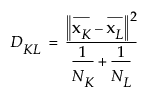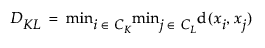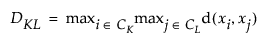Publication date: 05/24/2021

## Distance Method Formulas

This section provides the formulas used in calculating distances based on the Method that you select on the launch window. For a description of the methods, see Method for Distance Calculation.

The formulas use the following notation, where lowercase symbols generally pertain to observations and uppercase symbols to clusters:

n is the number of observations

v is the number of variables

xi is the ith observation

CK is the Kth cluster, subset of {1, 2,..., n}

NK is the number of observations in CK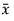is the sample mean vector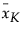is the mean vector for cluster CK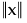is the square root of the sum of the squares of the elements of x (the Euclidean length of the vector x)

d(xi, xj) is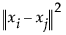The distance for the average linkage cluster method is: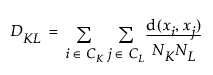Centroid Method

The distance for the centroid method of clustering is: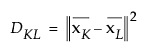Ward’s

The distance for Ward’s method is: